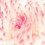# Infinite length Simple Pendulum

What is the time period of a simple pendulum of infinite length.

Can anyone explain this?Note by Selena Miller
7 years, 5 months ago

This discussion board is a place to discuss our Daily Challenges and the math and science related to those challenges. Explanations are more than just a solution — they should explain the steps and thinking strategies that you used to obtain the solution. Comments should further the discussion of math and science.

When posting on Brilliant:

• Use the emojis to react to an explanation, whether you're congratulating a job well done , or just really confused .
• Ask specific questions about the challenge or the steps in somebody's explanation. Well-posed questions can add a lot to the discussion, but posting "I don't understand!" doesn't help anyone.
• Try to contribute something new to the discussion, whether it is an extension, generalization or other idea related to the challenge.

MarkdownAppears as
*italics* or _italics_ italics
**bold** or __bold__ bold
- bulleted- list
• bulleted
• list
1. numbered2. list
1. numbered
2. list
Note: you must add a full line of space before and after lists for them to show up correctly
paragraph 1paragraph 2

paragraph 1

paragraph 2

[example link](https://brilliant.org)example link
> This is a quote
This is a quote
    # I indented these lines
# 4 spaces, and now they show
# up as a code block.

print "hello world"
# I indented these lines
# 4 spaces, and now they show
# up as a code block.

print "hello world"
MathAppears as
Remember to wrap math in $$ ... $$ or $ ... $ to ensure proper formatting.
2 \times 3 $2 \times 3$
2^{34} $2^{34}$
a_{i-1} $a_{i-1}$
\frac{2}{3} $\frac{2}{3}$
\sqrt{2} $\sqrt{2}$
\sum_{i=1}^3 $\sum_{i=1}^3$
\sin \theta $\sin \theta$
\boxed{123} $\boxed{123}$

Sort by:

Time period of a long pendulum is given by $\frac { 2\pi }{ \sqrt { \left( \frac { 1 }{ R } +\frac { 1 }{ l } \right) g } }$
for infinite pendulum 1/l will be neglected and the formula will be $2\pi \sqrt { \frac { R }{ g } }$
which on solving results in 86 mins

- 6 years, 2 months ago

bhai,how did you get this formula,proof??

- 4 years, 6 months ago

What is R? Also, what if the pendulum is in a gravity free region? Won't the friction of pendulum parts slow it down? Will the time period be different for the types of material we use to make bob and the pendulum?

- 4 years ago

R is the radius of the Earth.

In gravity free region the pendulum won't oscillate at all.

Friction will slow down all the pendulums whether infinite or not does not matter.

The time period does not depend on the bob if it is small.

- 4 years ago

Take any instant t let position at t=P join the mean position of the pendulums bob to the centre of the earth join P to the centre of the Earth Let angle between both lines be Q at P: mg acts vertically downwards mgsinQ provides restoring force F= -mgsinQ a=F/m= -gsinQ.........(1) for Q<10 degrees sinQ=Q(in radians) = tanQ let distance between the mean position and P be x tan Q=x/R where R is radius of earth a= -gx/R but a= -w^2 X x therefore w=underroot of g/R time period= 2pi/w therefore T= 2pi underroot (g/R) that is 86 Minutes

- 3 years, 6 months ago

it is because t=2pisqrtradius of earth/g

- 7 years, 5 months ago

You should consider a pendulum with the length L which is much longer than the Earth radius R. If you then use for the description of the pendulum motion the conservation law in the gravitational field (not in the uniform field), you will get the following result - angular frequency to the second power will be equal to g/R.

- 7 years, 5 months ago

You are right, it is just the change of g in the points close to the surface that makes the system oscilate harmonically. You should consider the change of the gravitational energy when the displacement is small and equate it to the change of kinetic energy. Finally in this particular case when the body is close to the surface of the Earth (in the strong limit L>>R) the period wouldn't depend on L.

- 7 years, 5 months ago

Ans is 84.6 minute

- 4 years, 5 months ago

t = 2 * pi * sqrt( l / g )

so i think if the its length is infinite so the time period also will be infinite :D

i don't know whether i am right or wrong :D

- 7 years, 5 months ago

Can someone please post a detailed proof??

- 5 years, 4 months ago

i have posted a detailed proof

- 3 years, 5 months ago

http://www.quantumstudy.com/physics/simple-harmonic-motion-12/ Check out here

- 3 years, 4 months ago

THE LENGTH OF THE PENDULUM IS MUCH LONGER THAN THE RADIUS OF THE EARTH SO IN THE FORMULA OF THE TIME PERIOD WE CONSIDER THE CHANGE IN THE VALUE OF g.

- 7 years, 5 months ago